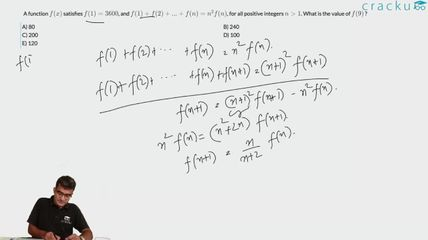Question 17

# A function $$f (x)$$ satisfies $$f(1) = 3600$$, and $$f (1) + f(2) + ... + f(n) =n^2f(n)$$, for all positive integers $$n > 1$$. What is the value of $$f (9)$$ ?

Solution

According to given conditions we get f(2)=f(1)/3 , then f(3)=f(1)/6, then  f(4)=f(1)/10 , then f(5)=f(1)/15 .

We can see the pattern here that the denominator goes on increasing from 3,3+3,6+4,10+5,15+6,.. so for the f(9) the denominator will be same as 15+6+7+8+9=45 .

So f(9)=3600/45 = 80

### View Video Solution• All Quant CAT Formulas and shortcuts PDF
• 30+ CAT previous papers with solutions PDF

##### Rohit Kumar

8 months, 4 weeks ago

It took me 5 minute to understood

##### SKRATCH PROOF

1 year, 3 months ago

it took me 45 min to understand it !

##### Rounak Lohia

3 years, 6 months ago

In the first line f(2)=f(1)/3